Page 7 of 7

### Re: User Functions Exchange

Posted: 06 Nov 2016 22:36
Wow, brilliant idea of using eval()!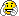You pass an expression as argument! Never seen this in my life.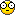However, I think the code you posted does not work. You reset \$list to nothing before it is processed by foreach. I changed the code by adding a separate variable \$listout and now it works:

Code: Select all

``````echo listFilter(get("list_recentlocations"), 'exists("{@Item}")', <crlf>)

/**
* List Filter
*
* @param {list} \$list              list of tokens
* @param {expression} \$filter      expression which returns {bool} on evaluation
*                                  placeholder: {@Item}
* @param {string} [\$separator='|'] list separator
* @param {flag} \$flag              + s : escape single quote (') for each item
*
* @return {list} filtered list of tokens
*/
function listFilter(\$list, \$filter, \$separator = '|', \$flag = '') {
\$listout = "";
foreach(\$item, \$list, \$separator) {
if (strpos(\$flag, 's') >= 0) {
\$item = replace(\$item, "'", "''");
}
\$expr = replace(\$filter, '{@Item}', \$item, 1);
if (eval(\$expr)) {
\$listout = \$listout . \$item . \$separator;
}
}
return trim(\$listout, \$separator, 'R');
}
``````

### Re: User Functions Exchange

Posted: 06 Nov 2016 22:53
admin wrote:Wow, brilliant idea of using eval()!You pass an expression as argument! Never seen this in my life.However, I think the code you posted does not work. You reset \$list to nothing before it is processed by foreach. I changed the code by adding a separate variable \$listout and now it works:
ThanksI just realize the typo. Originally the code is function listFilter(\$ListOfToken, { ... }. Then, I feel that \$ListOfToken is too long ...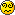### Re: User Functions Exchange

Posted: 07 Nov 2016 06:17
admin wrote:Never seen this in my life.Usually you have objects for that (e.g. Comparators).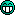### Re: User Functions Exchange

Posted: 15 Mar 2017 19:20
gethtmltoken(\$url, \$option, [\$index]) : returns option value or optionlist in an html() GET form submit url.
Uses the regexescape() as <xyscripts>\_inc\regexescape.xyi.

function

Code: Select all

``````INCLUDE  "_inc\regexescape.xyi";
FUNCTION gethtmltoken(\$url, \$option, \$index=1) {
/* returns option value or optionlist in an html() GET form submit url
** \$url      the url string to parse
** \$option   the option's value to return
** \$index    number: 1-based index of option in multiples of same name
**           "list": lists existing options in order, each on one line
** NOTE: there's ambiguity between non-existing option and empty value
*/
\$url     = trim(\$url, "?", "L");  // strip starting ? char
\$url     = "&" . \$url;  // ease parsing - now all tokens starts with &
if (\$index == "list") {
\$options = regexmatches(\$url, "\&[^=]*", <crlf>);
\$options = utf8decode(urldecode(replace(\$options, '&', '')));
return \$options;
}
\$options = regexmatches(\$url, "\&" . regexescape(\$option) . "=[^&]*");
\$option  = gettoken(\$options, \$index, "|");
\$value   = gettoken(\$option, 2, "=");
\$value   = utf8decode(urldecode(\$value));
return \$value;
}
``````
call
assuming the functions gethtmltoken() is saved in <xyscripts>\_inc\gethtmltoken.xyi

Code: Select all

``````INCLUDE "_inc\gethtmltoken.xyi";
// \$html = html('<html>...<form method="GET">...</html>');
\$html = "?type=expert&flags=29&pattern=^.*%26(\\)%24&go=ok";
text gethtmltoken(\$html,'pattern');  // ^.*&(\\)\$
text gethtmltoken(\$html,, 'list');
/* return:
type
flags
pattern
go
*/
``````

### Re: User Functions Exchange

Posted: 06 Jun 2017 19:40
getitemindex()

Code: Select all

``getitemindex([\$items=<crlf>-separated current selection], [\$sep=<crlf>])``
Returns list indexes of given items in current list.

function code

Code: Select all

``````INCLUDE  "_inc\getitemindex.xyi";
FUNCTION getitemindex(\$items=<selitems <crlf>>, \$sep=<crlf>) {
/* Returns indexes of given items in current list
** \$items    full paths to one or more items, separated by \$sep
**           default: <crlf>-separated current selection
** \$sep      separator in \$items list and returned index list
**           default: <crlf>
** 0 is returned for items that are not in current list.
*/
\$list   = listpane(,,, <crlf>);
\$return = '';
foreach (\$item, \$items, \$sep) {
\$index  = gettokenindex(\$item, \$list, <crlf>);
\$return = \$return . \$sep . \$index;
}
\$return = substr(\$return, strlen(\$sep));
return  \$return;
}

``````
calling example code
assuming the functions getitemindex() is saved in <xyscripts>\_inc\getitemindex.xyi

Code: Select all

``````INCLUDE "_inc\getitemindex.xyi";
goto <xypath>;
echo getitemindex(<xy> . <crlf> . get('help')); // indexes of xyplorer.exe and xyplorer.chm, eg, 11<crlf>9
``````

### Re: User Functions Exchange

Posted: 01 Oct 2017 17:32
A tiny useless utility function.

flip(\$var): toggle \$var between 0/""/False and 1/True.

code

Code: Select all

``FUNCTION flip(\$var){ return !\$var; }``
code (variable passes by reference)

Code: Select all

``FUNCTION flip(&\$var){ \$var = !\$var; return \$var; }``
example

Code: Select all

``````INCLUDE "_inc\flip.xyi";
\$a = 1; echo flip(\$a); set \$b; echo flip(\$b);``````

### Re: User Functions Exchange

Posted: 01 Oct 2017 22:05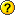Seems the description is wrong: it doesn't change (i.e. flip) anything: the variable stays as it is.
It just returns the binary opposite, i.e. NOT.

So just use

Code: Select all

``   \$a = 1; echo ! \$a; set \$b; echo !\$b;``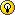### Re: User Functions Exchange

Posted: 02 Oct 2017 09:10
yeah, so there's a variation now. This is nothing serious. Just a beautifier around the ! expression.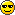### Re: User Functions Exchange

Posted: 02 Oct 2017 10:57
Yes, NOT !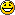### Re: User Functions Exchange

Posted: 02 Dec 2018 11:01
xycolorconv
a set of complementary functions that convert between regular hex colors and the decimal color format used in XYplorer.ini.

function code

Code: Select all

``````/* xycolorconv.xyi
convert between Xyplorer's int and regular hex color representation.
*/
NAMESPACE xycolorconv

/* === helper functions === */

FUNCTION normalize_int(\$int) {
assert \$int == \$int+0;
assert \$int >= 0;
assert \$int <= hextodec('FFFFFF');
// assert \$int == round(\$int);
\$int = round(\$int);
return \$int;
}

FUNCTION normalize_hex(\$hex) {
\$hex = replace(\$hex, '#', 5:=1);
assert strlen(\$hex) <= 6;
assert !(regexmatches(\$hex,'[^0-9a-f]'));
return strrepeat('0', 6 - strlen(\$hex)) . \$hex;
}

FUNCTION flip_hex(\$hex) {
return substr(\$hex, 4, 2) . substr(\$hex, 2, 2) . substr(\$hex, 0, 2);
}

/* === main converters === */

FUNCTION to_hex(\$int) {
\$int = normalize_int(\$int);
\$hex_bgr = '';
while (\$int > 0) {
\$div = \$int / 16;
\$int = floor(\$div);
\$remainder = \$div - \$int;
\$hex = \$remainder * 16;
\$hex = replacelist(\$hex, '10|11|12|13|14|15', 'A|B|C|D|E|F', '|');
\$hex_bgr = \$hex . \$hex_bgr;
}
\$hex_bgr = normalize_hex(\$hex_bgr);
\$hex_rgb = flip_hex(\$hex_bgr);
return \$hex_rgb;
}

FUNCTION to_int(\$hex) {
\$hex_rgb = normalize_hex(\$hex);
\$hex_bgr = flip_hex(\$hex_rgb);
\$int = hextodec(\$hex_bgr);
return \$int;
}

``````
example:
assuming the complete code above is saved as "<xyscripts>/_inc/xycolorconv.xyi":

Code: Select all

``````INCLUDE "_inc/xycolorconv.xyi";
"colorconv"
echo xycolorconv::to_hex(3727104); // convert to hex color
echo xycolorconv::to_int(FE98B0); // convert to <xyini> decimal color``````